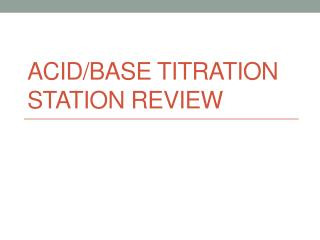DownloadDownload PresentationAcid/Base Titration Station Review

# Acid/Base Titration Station Review

Télécharger la présentation## Acid/Base Titration Station Review

- - - - - - - - - - - - - - - - - - - - - - - - - - - E N D - - - - - - - - - - - - - - - - - - - - - - - - - - -
##### Presentation Transcript

1. Acid/Base Titration Station Review

2. As you come in, • The Materials: • Remote control, paper, pencil, and calculator for quiz • Paper, pencil, calculator for Titration Stations • The Plan: • Answer questions about pH, pOH, and Kw • Take pH, pOH, and Kw Quiz • Solve the Titration Stations • Check our Titration Station answers • The Assessment: • Titration Quiz – TOMORROW • Cumulative Quiz (Solutions) – Wednesday • Unit Ten Acid/Base Test – Thursday • Cumulative Quiz (A/B) - Tuesday

3. Station One • 100-mL of HBr solution reacts with 250-mL of Mg(OH)2 solution.  If the pH of the base is 12.9, what molarity of the acid is needed to react with the base? • 0.397 M HBr

4. Station Two • In a titration lab, a student slowly adds 18.3mL of 0.115M sodium hydroxide from a buret to 25mL of nitric acid in an Erlenmeyer flask to neutralize the acid. What is the molarity of the acid? • 0.084 M

5. Station Three • Titration reveals that 11.6 mL of 3.0 M sulfuric acid are required to neutralize the sodium hydroxide in 25.00 mL of NaOH solution. What is the molarity of the NaOH solution? • 2.78 M

6. Station Four • How many mL of a 3M NaOH solution are required to completely neutralize 20.0 mL of 1.5M H2SO4? • 20 mL

7. Station Five • What volume of 0.25M phosphoric acid is required to neutralize 30.0mL of 0.05M barium hydroxide? • 4 mL

8. Station Six • Calculate the mass of aluminum hydroxide required to completely react with 20.0 mL of 0.45M HCl. • 0.234 grams